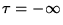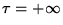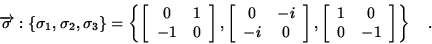Next: SUMMARY Up: ACCELERATION-INDUCED CARRIER OF THE Previous: Geometry of the Space

## Quantum Mechanical Carrier of Gravitational Imprints

Our proposal is to have each spinor field serve as a carrier of the imprints of gravitation: A gravitational disturbance confined to, say, Rindler I or II would leave its imprint on a spinor field atby changing it into another spinor field at.

We know that in the absence of gravitation each of the positive and negative Minkowski plane wave solutions evolves independently of all the others. This scenario does not change under Lorentz boosts and spacetime translations. This is another way of saying that the system described by these solutions is Poincare invariant. Will the proposed carriers comply with this invariance, which is stipulated by fundamental requirement 3.? To find out, consider a typical plane wave. Its spinor representation (3) is10

This is a state with 100% correlation between the boost energy and the polarization (spin'') degrees of freedom. In recent years such states have been called entangled'' states. Suppose that for each boost energy we determine the normalized Stokes parameters of this polarization, i.e. the expectation values of the three modified Pauli spin'' matrices11

This is a three-dimensional vector field over the base manifold R , and is given by12

In compliance with requirements 2. and 3., this vector field is (a) independent of the particle mass and (b) the same for all positive (negative) Minkowski plane wave modes, a fact which expresses its Poincare invariance. The presence of gravitation would leave its imprints by producing characteristic alterations in this vector field.Next: SUMMARY Up: ACCELERATION-INDUCED CARRIER OF THE Previous: Geometry of the Space
Ulrich Gerlach
1998-02-20